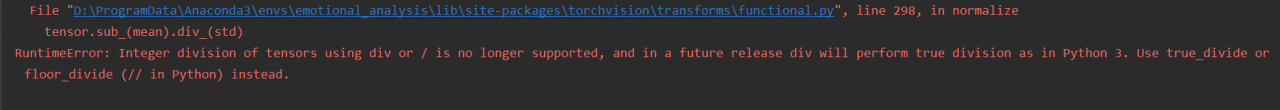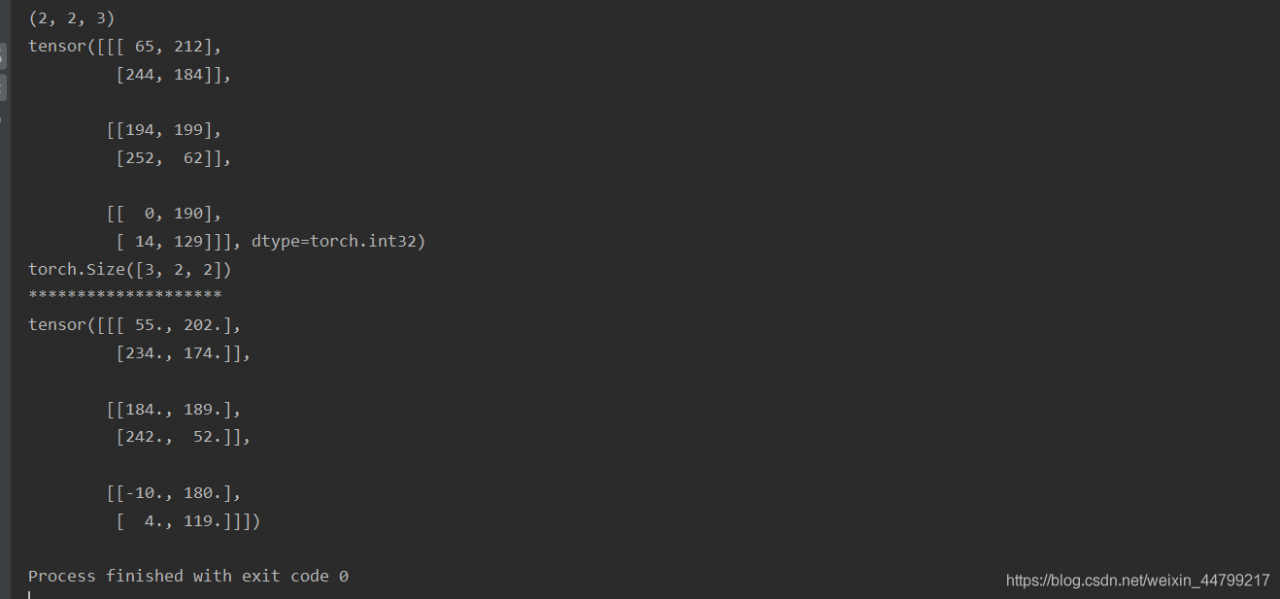# RuntimeError: Integer division of tensors using div or / is no longer supported, and in a future rel

RuntimeError: Integer division of tensors using div or/is no longer supported, and in a future release div will perform true division as in Python 3. Use true_divide or floor_divide (// in Python) instead.

``````from torchvision import transforms
import numpy as np

data = np.random.randint(0, 255, size=12)
img = data.reshape(2, 2, 3)
print(img.shape)
img_tensor = transforms.ToTensor()(img)  # Convert to tensor
print(img_tensor)
print(img_tensor.shape)
print("*" * 20)
norm_img = transforms.Normalize((10, 10, 10), (1, 1, 1))(img_tensor)  # Perform normative processing
print(norm_img)
``````

Operation effect:reason:

Pytorch1.5.0 is OK, but when upgrading to 1.6.0, it is found that division between tenor and int cannot be directly performed with ‘/’.

Solution:

Standardize the data processing

Example code:

``````from torchvision import transforms
import numpy as np

data = np.random.randint(0, 255, size=12)
img = data.reshape(2, 2, 3)
print(img.shape)
img_tensor = transforms.ToTensor()(img)  # convert to tensor
print(img_tensor)
print(img_tensor.shape)
print("*" * 20)
img_tensor = img_tensor.float()  # Add this line
norm_img = transforms.Normalize((10, 10, 10), (1, 1, 1))(img_tensor) # Perform normalization
print(norm_img)
``````

Results of operation: